#### You may also like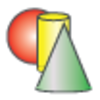### Sponge Sections

You have been given three shapes made out of sponge: a sphere, a cylinder and a cone. Your challenge is to find out how to cut them to make different shapes for printing.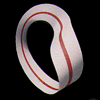### Bands and Bridges: Bringing Topology Back

Lyndon Baker describes how the Mobius strip and Euler's law can introduce pupils to the idea of topology.### Triangles in the Middle

This task depends on groups working collaboratively, discussing and reasoning to agree a final product.

# 3D Stacks

##### Age 7 to 14 Challenge Level:

This activity may be used as a follow-on for those who have successfully worked at Doplication.

In this example of a 2 by 3 by 4 we see in the red balls, as two layers of 3 by 4.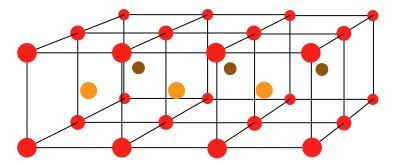These two layers together can also be viewed as representing 6 cubes.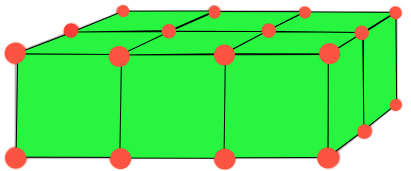In the top picture the centre of each cube is shown with a light/dark brown ball.

In Doplication you would have had;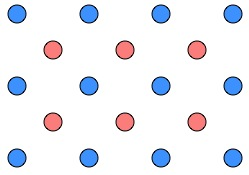which we called $4$ ? $3$.

In this activity it would be called something different involving $4, 3, 2$. Just for now I am going to use @, so the diagram above represents $4$@$3$@$2$.

I invite you to explore many such arrangements and find a way of recording your results.
You might like to just look at the arrangements that are like cubes - $3$@$3$@$3$ , $4$@$4$@$4$, $5$@$5$@$5$, etc.

As with most mathematics it's good to compare so maybe compare the results for squares in Doplication and cubes in 3D Stacks.